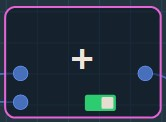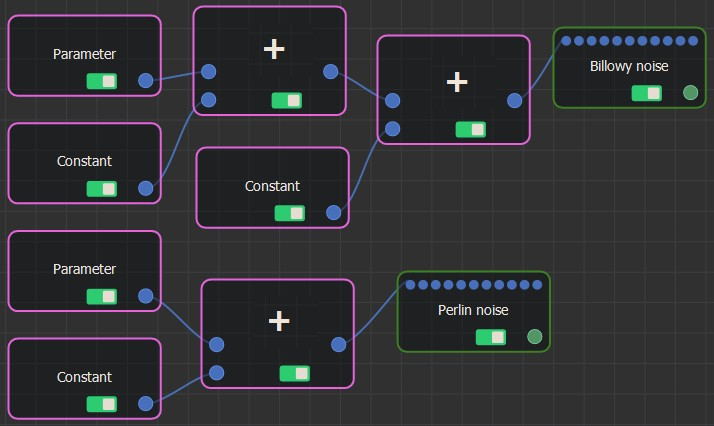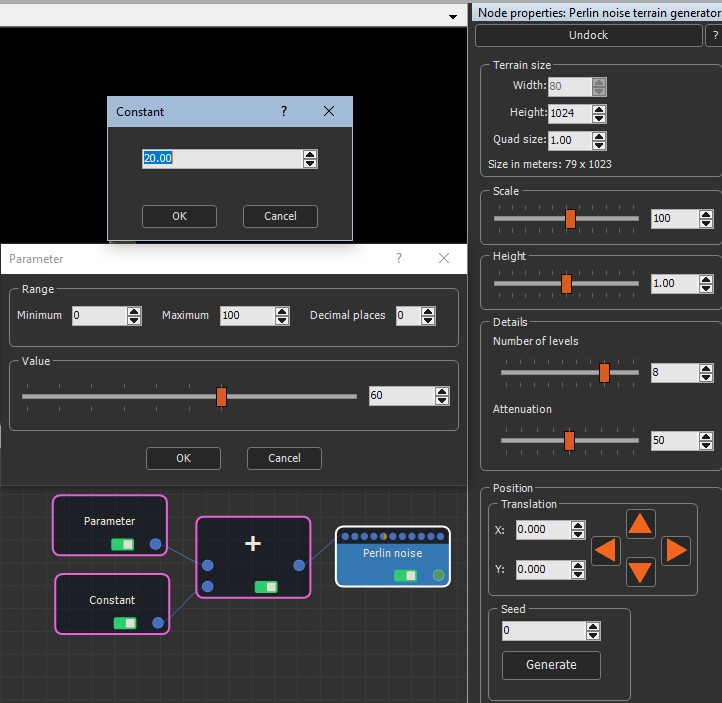### Adding a Plus node

The Plus node adds two input numbers to generate an output number. It is an alternative to the Formula node, but allows you to code a formula as a graph rather than a formula.

It has two input connectors and one output connector and adds the first input value to the second input value.

To add a Plus node, select Edit in the main menu or right-click in the Graph Editor and select Create Node  > Calculation  > Plus.The Plus node has no parameters.

### Linking a Plus node

The Plus node input connectors link to two input Calculation nodes and the output connector to a Calculation node or an optional connector.### Example

In the example below, the Parameter value and a Constant value are summed to drive the terrain size of the Perlin noise. Note that the Terrain size value in the Perlin noise parameters is grayed out because it is replaced by the summed values.Copyright © 2021 · All Rights Reserved · Wysilab